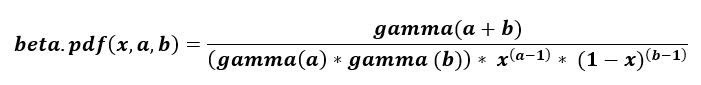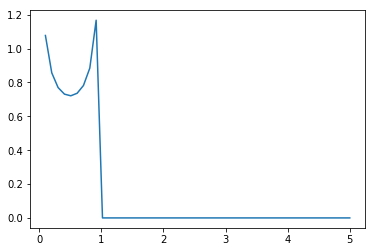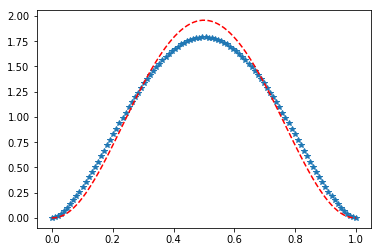Skip to content
Related Articles
scipy stats.beta() | Python
• Last Updated : 20 Mar, 2019

scipy.stats.beta() is an beta continuous random variable that is defined with a standard format and some shape parameters to complete its specification.Parameters :
q : lower and upper tail probability
a, b : shape parameters
x : quantiles
loc : [optional] location parameter. Default = 0
scale : [optional] scale parameter. Default = 1
size : [tuple of ints, optional] shape or random variates.
moments : [optional] composed of letters [‘mvsk’]; ‘m’ = mean, ‘v’ = variance, ‘s’ = Fisher’s skew and ‘k’ = Fisher’s kurtosis. (default = ‘mv’).

Results : beta continuous random variable

Code #1 : Creating beta continuous random variable

 `# importing scipy``from` `scipy.stats ``import` `beta`` ` `numargs ``=` `beta.numargs``[a, b] ``=` `[``0.6``, ] ``*` `numargs``rv ``=` `beta(a, b)`` ` `print` `(``"RV : \n"``, rv)`

Output :

```RV :
<scipy.stats._distn_infrastructure.rv_frozen object at 0x0000029482FCC438>
```

Code #2 : beta random variates and probability distribution function.

 `import` `numpy as np``quantile ``=` `np.arange (``0.01``, ``1``, ``0.1``)``  ` `# Random Variates``R ``=` `beta.rvs(a, b, scale ``=` `2``,  size ``=` `10``)``print` `(``"Random Variates : \n"``, R)`` ` `# PDF``R ``=` `beta.pdf(quantile, a, b, loc ``=` `0``, scale ``=` `1``)``print` `(``"\nProbability Distribution : \n"``, R)`

Output :

```Random Variates :
[1.47189604 1.47284574 1.84692416 1.0686604  0.32709236 1.96857076
0.00639731 1.97093898 1.34811881 0.34269426]

Probability Distribution :
[2.62281037 1.04883674 0.84934164 0.76724957 0.73040985 0.72096547
0.73529768 0.77903762 0.8752367  1.1264383 ]```

Code #3 : Graphical Representation.

 `import` `numpy as np``import` `matplotlib.pyplot as plt`` ` `distribution ``=` `np.linspace(``0``, np.maximum(rv.dist.b, ``5``))``plot ``=` `plt.plot(distribution, rv.pdf(distribution))`

Output :Code #4 : Varying Positional Arguments

 `from` `scipy.stats ``import` `arcsine``import` `matplotlib.pyplot as plt``import` `numpy as np`` ` `x ``=` `np.linspace(``0``, ``1.0``, ``100``)`` ` `# Varying positional arguments``y1 ``=` `beta.pdf(x, ``2.75``, ``2.75``)``y2 ``=` `beta.pdf(x, ``3.25``, ``3.25``)``plt.plot(x, y1, ``"*"``, x, y2, ``"r--"``)`

Output :Attention geek! Strengthen your foundations with the Python Programming Foundation Course and learn the basics.

To begin with, your interview preparations Enhance your Data Structures concepts with the Python DS Course. And to begin with your Machine Learning Journey, join the Machine Learning – Basic Level Course

My Personal Notes arrow_drop_up